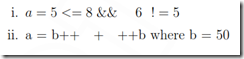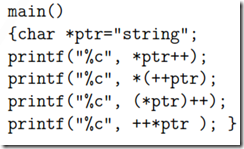### Fundamentals of Computing and Programming (FOC)–May / June 2010 Question Paper

Anna University

B.E./B.Tech. DEGREE EXAMINATIONS, MAY/JUNE 2010

Regulations 2008

First Semester

Common to all branches

GE2112 Fundamentals of Computing and Programming

Time: Three Hours

Maximum: 100 Marks

Part A - (10 x 2 = 20 Marks)

1. What a re Mainframe computers? Give t he name of any one Mainframe computer.

2. Convert 0.4375 decimal to binary system.

3. Differentiate between hardware and software.

4. What do you mean by URL? Mention its uses.

5. Write down steps involved in writing a program to solve a problem.

6. Define the term Assembler

7. List out the rules to be followed i n forming a n identifier.

8. Write a C program for the following expressions.9. What do you mean by a pointer? Mention its uses..

10. Determine the output for the following printf statementsPart B - (5 x 16 = 80 Marks)

11. (a) Explain in detail t he evolution of computers. (16)

OR

11. (b) (i) How does one specify t he configuration of a computer system? Give t he signifi- cance of each term in it. (6)

(ii) Convert t he hexadecimal number A5B to octal number.Explain each step involved in t he conversion. (10)

12. (a) (i) What is system software? Explain various system software i n detail. (8) (ii) Briefly explain t he steps i n software development. (8)

OR

12. (b) (i) What is an internet? Explain its evolution a nd its services (8)

(ii) Explain how internet connection can be established? (8)

13. (a) (i) Write down the algorithm to find th e largest number among three given numbers and outline t he steps i n the algorithm with th e inputs 5, 17, 3. (8)

(ii) Draw flowchart to compute the salary of a n employee i n a company. Assume that there a re two types of employees in t he company daily wages and regular. Salary is calculated as number of hours worked* wages per hour for daily wagers and basic pay +(% of DA * basic pay)/100 + HRA + medical allowance for regular employees. Sketch the flow of your design for a regular employee with basic pay = 5000, % of DA = 75 % a nd HRA = 500. (8)

OR

13. (b) (i) What is pseudo code? Explain how it can be designed a nd its benefits a nd limitations. (8)

14. (a) (i) Develop a C program that computes and prints a table of factorials for a given integer (8)

(ii) Describe the features of different data types i n C (8) OR

14. (b) (i) Explain briefly t he formatted a nd unformatted I/O functions in C. (8)

(ii) Write a C program to evaluate the following series.

15. (a) (i) Briefly explain the various string handling functions in C. (4) (ii) What do you mean by call by reference? Write a program i n C to exchange t he value of two variables using call by reference. (6)

(iii) Write a C program to find the largest an d smallest number in t he given array.(6)

OR

15. (b) (i) Write a C program to find th e factorial of a given number using recursion. (6)

(ii) Write a C program to accept records of 20 states using array of structures. The structure should contain name of the state and number of engineering colleges, medical colleges, management colleges, science colleges. Calculate a nd display the total colleges i n each state and th e state which is having highest number of colleges. (10)